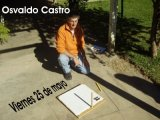March 2007

Flag City Country School Latitude (°) +N/-S Longitude (°) +E/-W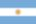Cañada de Gómez Argentina Centro Interactivo de Ciencia y Tecnología -32.817 -61.400
March 18 cm cm -33°12’
March 20 cm cm -30°04’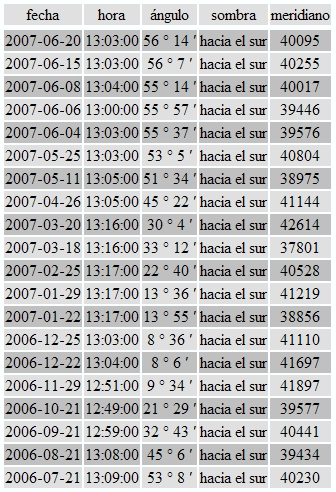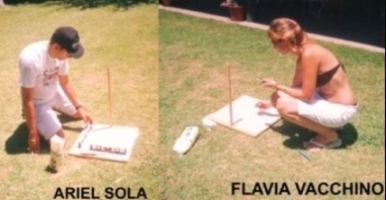Before... and after noon...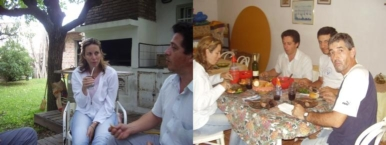When begun the campaign 2006-2007, I was several months without other measurements from partners, to calculate the terrestrial meridian. To solve this problem, I used Cooper formula (1969):
Declination = - 23.45 sin (2*PI* (d+284) /365
Where d is the day of the year.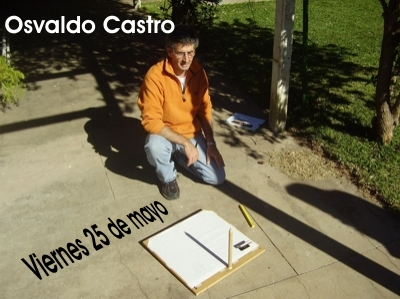In order compute the distance between our parallel and the one of another city located in another meridian , we used formulate:
d = (111.3 km) · arcos ( sin a · sin b + cos a . cos b cos · cos(c- e))
Where a and b they are the latitudes of both cities, c and e are the longitudes. But that formula delivers the distance between cities; that does not interest to us: The reason is that c=e (both in he himself meridian, and different parallel), thus: d = (111.3 km) · arcos ( sin a · sin b + cos a . cos b )
We are interested by the ditance to the equator, so:
a=0 and b= our latitude (or yours).

d = (111.3 km). arccos (cos b) = (111.3 km). b
We took 111.3 for a circumference from 40075.2 km.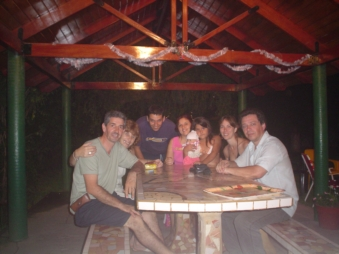When there is no sun...

Home page | Contact | Site Map | | Statistics | visits: 159933